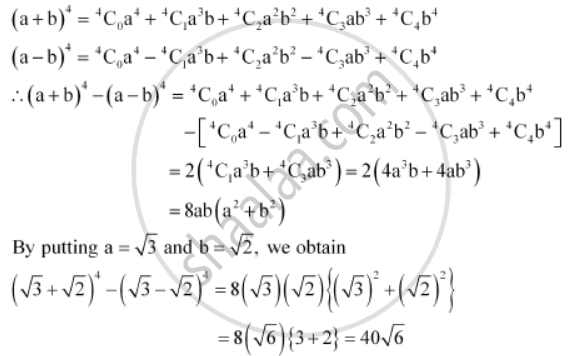CBSE (Commerce) Class 11CBSE
Share

Find (A + B)4 – (A – B)4. Hence, Evaluate (Sqrt3 + Sqrt2)^4 - (Sqrt3 - Sqrt2)^4 - CBSE (Commerce) Class 11 - Mathematics

ConceptBinomial Theorem for Positive Integral Indices

Question

Find (a + b)4 – (a – b)4. Hence, evaluate (sqrt3 + sqrt2)^4 - (sqrt3 - sqrt2)^4

Solution 1

Using Binomial Theorem, the expressions, (a + b)4 and (a – b)4, can be expanded asSolution 2

Using Binomial Theorem, the expressions, (a + b)4 and (a – b)4, can be expanded asIs there an error in this question or solution?

Video TutorialsVIEW ALL 

Solution Find (A + B)4 – (A – B)4. Hence, Evaluate (Sqrt3 + Sqrt2)^4 - (Sqrt3 - Sqrt2)^4 Concept: Binomial Theorem for Positive Integral Indices.
S